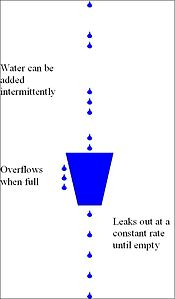long timeStamp=getNowTime();
int reqCount=0;
const int maxReqCount=10000;//时间周期内最大请求数
const long effectiveDuration=10;//时间控制周期

bool grant(){
long now=getNowTime();
if (now <timeStamp+effectiveDuration){//在时间控制范围内
reqCount++;
return reqCount>maxReqCount;//当前时间范围内超过最大请求控制数
}else{
timeStamp=now;//超时后重置
reqCount=0;
return true;
}
}（图来自wikipedia）

long timeStamp=getNowTime();
int capacity;        // 桶的容量
int rate ;          //水漏出的速度
int water;          //当前水量

bool grant() {
//先执行漏水，因为rate是固定的，所以可以认为“时间间隔*rate”即为漏出的水量
long  now = getNowTime();
water = max(0, water- (now - timeStamp)*rate);
timeStamp = now;

if (water < capacity) { // 水还未满，加水
water ++;
return true;
} else {
return false;//水满，拒绝加水
}
}


long timeStamp=getNowTime();
int capacity;              // 桶的容量
int rate ;              //令牌放入速度
int tokens;            //当前水量

bool grant() {
//先执行添加令牌的操作
long  now = getNowTime();
tokens = max(capacity, tokens+ (now - timeStamp)*rate);
timeStamp = now;
//令牌已用完，拒绝访问
if(tokens<1){
return false;
}else{//还有令牌，领取令牌
tokens--;
retun true;
}
}